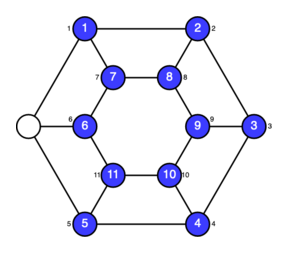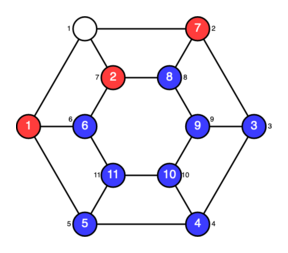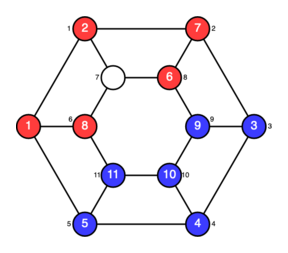## Slide/Swap on Cubic Graphs

Slide/Swap is a scrambly puzzle where you slide tiles from one space to another. The rules are simple:
• You can slide a tile along an edge into the empty space, but...
• ...whenever you slide a tile, its two neighboring tiles will trade places too.

Choose a board to play on, hit the "Scramble" button, and see if you can get all the tiles back to their starting spots. Even the small boards are frustrating at first, but you'll get the hang of it!Start Playing!

### A Little Background

Slide/Swap is a descendent of two other scrambly puzzles:
1. The well-known 15 Puzzle, and
2. The lesser-known Conway M13 Puzzle (which is only briefly described at the Wikipedia link; see the References below for more information.)

Simple sliding games like the 15 Puzzle, with no swap, can be played on the vertices of any graph.

M13 has a "slide-and-swap" type rule, but the way it is defined is very specific to one particular playing board, a projective plane of order 3. It does not generalize in any obvious way to a family of games on larger projective planes or other combinatorial structures.

Slide/Swap keeps the frustrating complexity of the M13 swap rule, but it can be played on any graph where each vertex has exactly three neighbors. These are called cubic or 3-regular graphs. Cubic graphs are plentiful, so this affords some variety (and escalation in difficulty). It also raises some interesting mathematical questions.

### The 8-Vertex Cube Graph

If you have had a first course in group theory, you may know that the smallest nonabelian simple group is A5, the alternating group of 60 elements. It is the rotational symmetry group of the dodecahedron and the icosahedron.

Slide/Swap on the 8-vertex cube graph gives rise to the next smallest nonabelian simple group, which has 168 elements. You might know it as a group of invertible 3×3 matrices (mod 2), but it isn't the symmetry group of any solid you can hold in your hands. Slide/Swap is a nice way to get a concrete feel for it.

The linear algebra is still there, though. If you label the vertices of the cube correctly, you will see that it is a vector space and, interpreted correctly, slide/swap moves are linear transformations. This is peculiar to the cube, and other graphs do not behave this way. There's a full explanation in the Slide-and-swap Permutation Groups article in the references below.

1. A Modern Treatment of the 15 Puzzle (Archer, 1999)

A little history of the 15 Puzzle, and a proof of the fact that all even permutations of the tiles can be achieved.

2. Graph Puzzles, Homotopy, and the Alternating Group (Wilson, 1974)

The classic (but somewhat difficult) paper which studies the generalization of the 15-puzzle to arbitrary graphs.

3. Slide-and-swap Permutation Groups (Ekenta, Jang, and Siehler, 2014)

This was joint work with two summer research students. We obtain a result for Slide/Swap similar to the central theorem in Wilson's article, and we explain why the cube graph is such an interesting exception.

4. Conway's Puzzle M13 (A blog post from 2007)

As far as I can remember, this was my first introduction to M13.

5. Depth and Symmetry in Conway's M13 Puzzle (Siehler, 2011)

My exposition of M13, including a solution method and some theorems about the relationship between the geometry of the projective plane and the permutations of the tiles in the game

6. The Mathieu group M12 and its pseudogroup extension M13 (Conway, Elkies, and Martin, 2006)

A much more substantial dive into the mathematics of M13 (which inspired my simpler article above)